# Acta Universitatis Danubius. Œconomica, Vol 13, No 1 (2017)

Monetary Policy in a DSGE New Keyesian Model –Case Study for Romania

Georgiana Alina Ionita1

Abstract: The paper proposes the analysis of a Basic New Keynesian model with imperfect competition in goods market and price adjustment mechanism for the macroeconomic context of Romania, as an emerging country. Given the vulnerabilities of the economy of Romania at the beginning and during the recent global economic and financial crisis, there is an increased interest to identify models that can explain the main features of Romania macroeconomic data and to put an eye on shocks that are really necessary to describe the stochastic dynamic of macroeconomic variables.

Keywords: monetary policy; exogenous; shocks

JEL Classification: C01; D50; B22; C4

1. Introduction

The model proposed by the current working paper has the purpose to analyze the impact of exogenous shocks to the macroeconomic variables, in the context of conducting a monetary policy rule through a Taylor rule based on targeting inflation and output gap.

The paper is focused on the analysis of impulse response functions in the context of uncertainty affecting the stochastic behavior of macroeconomic variables, through the following shocks: monetary policy shock, technology shock and preference shock, meanwhile conducting monetary policy.

In addition, another objective of the article is to analyze the suitability of the model for the macroeconomic context of Romania, through the following statistics: autocorrelation coefficients, Blanchard-Kahn stability test, variance decomposition and the economic interpretation of impulse response functions and orthogonalized shocks in relation with the empirical macroeconomic evidence.

2. The Model

The model assumes imperfect competition of good, as firms produce different goods for which a part of firms they set price, while other keep the price unchanged, as in the article proposed by Gali, J. (2015).

The agents assumed by the model are: household, firms and Central Bank, as the authority responsible with the monetary policy.

2.1. Household

Households maximize the following utility function: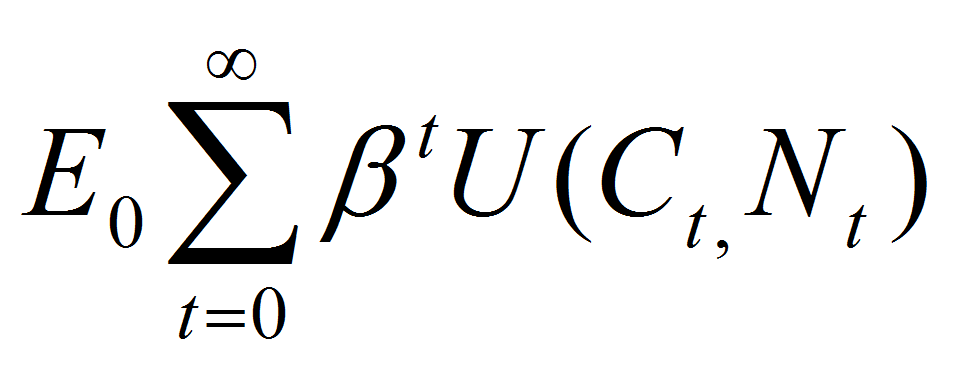,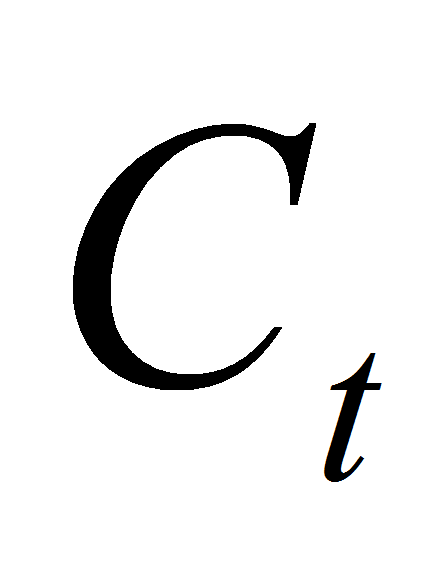representing consumption index described as follows: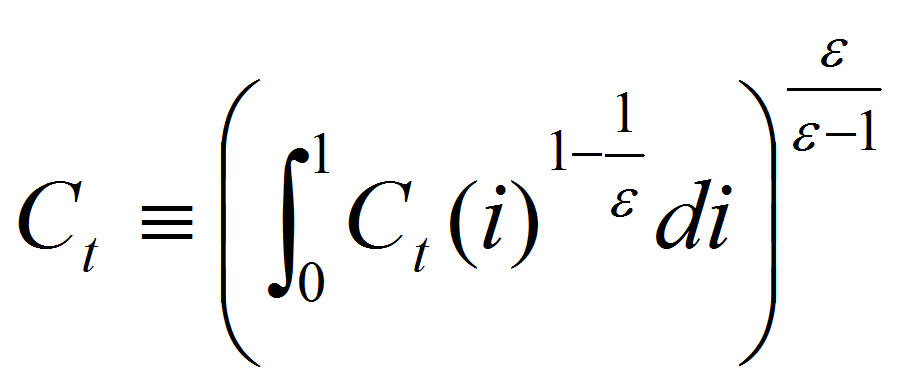in relation with the consumption of good i,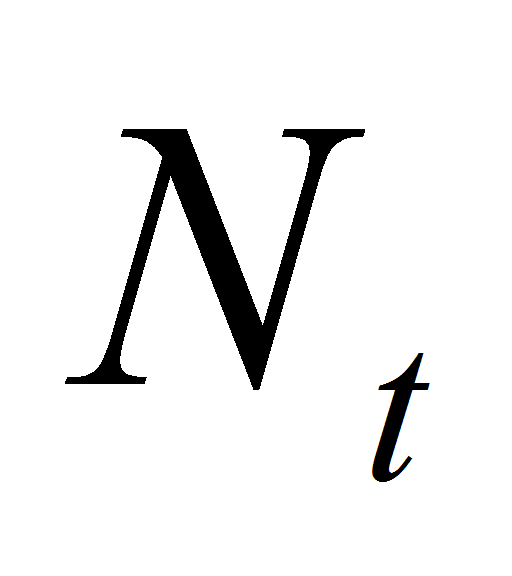represents the number of hours worked andrepresent the discount factor.

Households are also subject to the following budget constraint: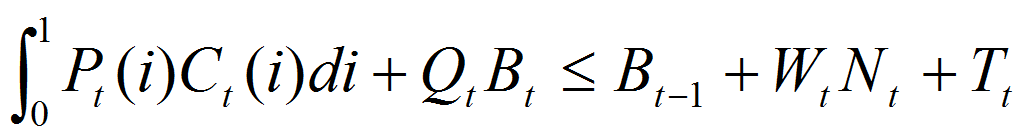, where: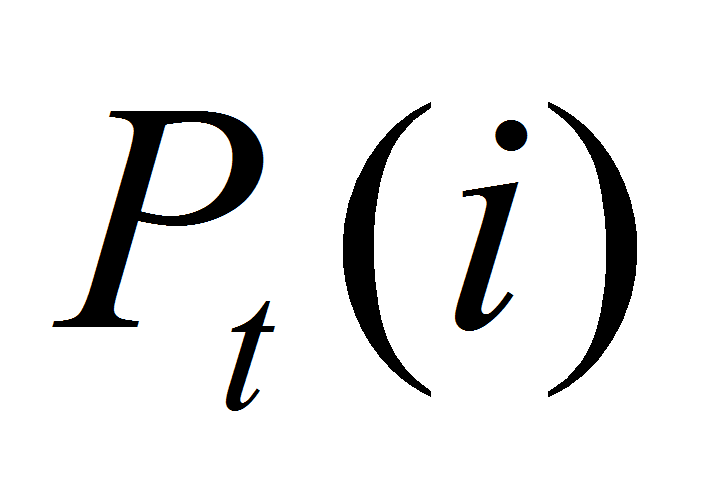is the price of good i at moment “t”,= index of hours worked at moment “t”,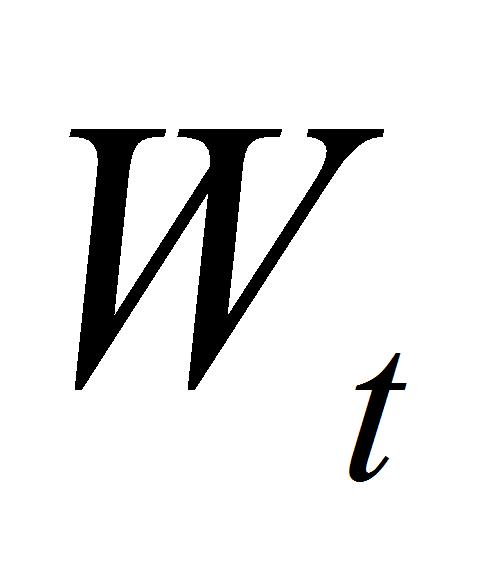is nominal wage at moment “t”,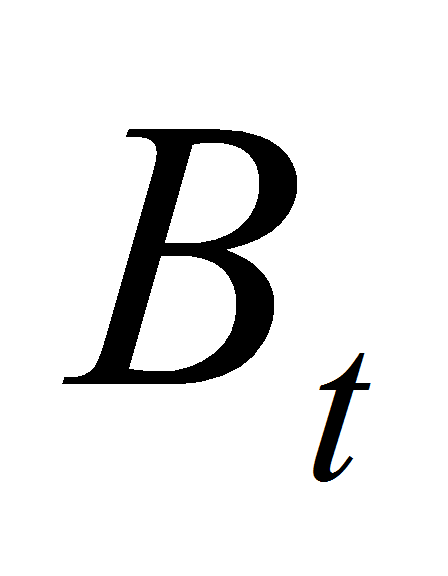represents acquired one period bond at moment “t”,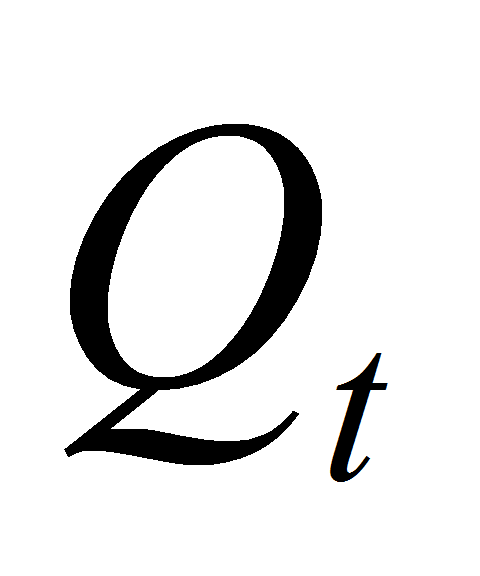is the price of bond acquired at moment “t” and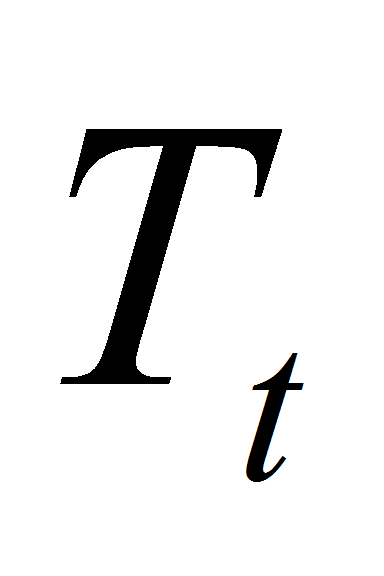= lump-sum income component at moment “t”. The demand equation is described by the equation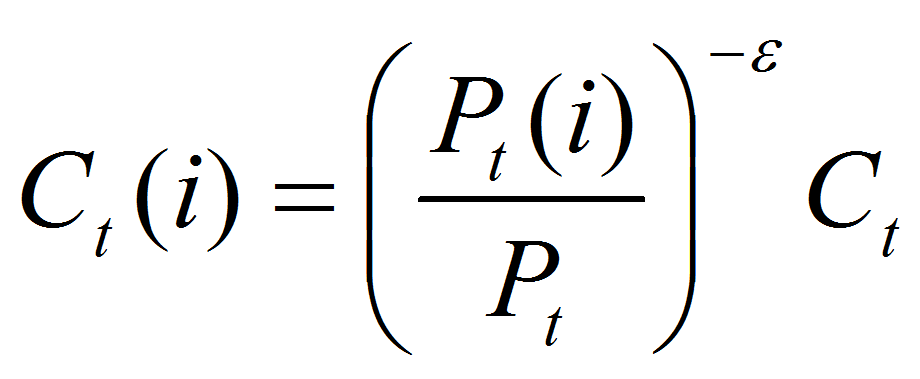, where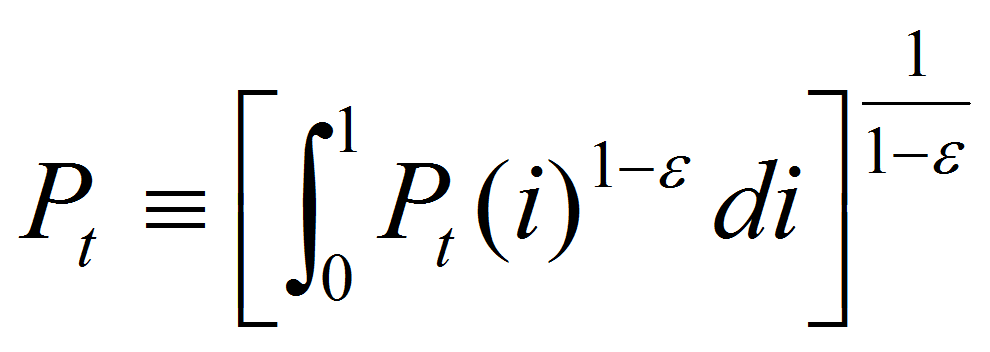is the aggregate price index. The optimal condition for consumers behavior is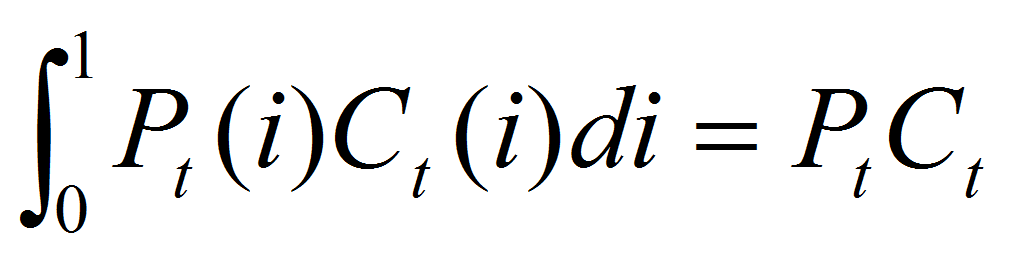. The resulting budget constraint for consumers is described as follows: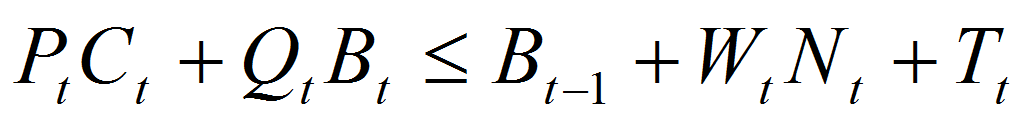.

The optimal consumption /savings and labour supply decisions are described by the following utility ratio: -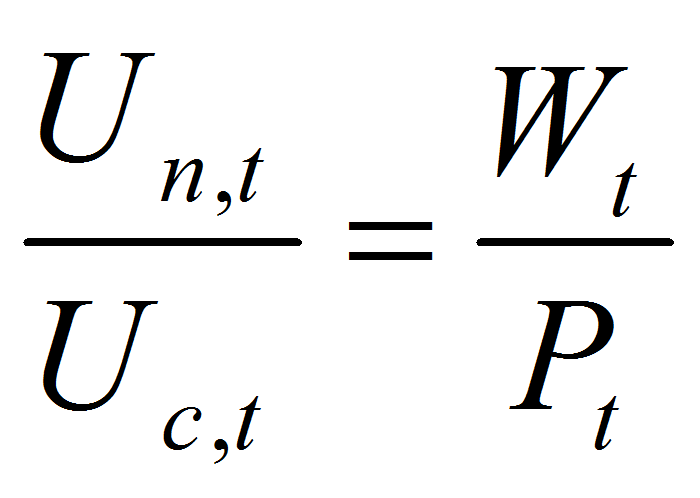;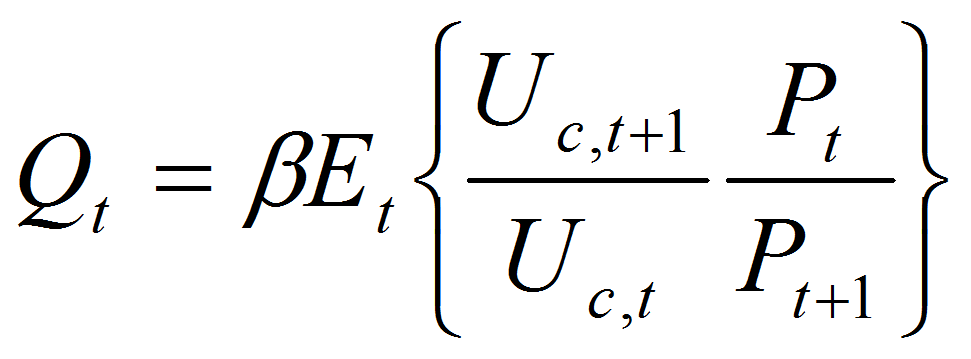.

The utility function for one period is described as follows: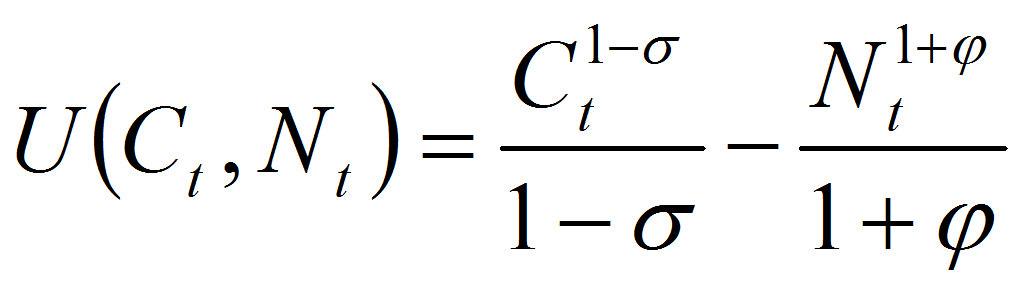.

2.2. Firms

The production function described by the firms is as follows: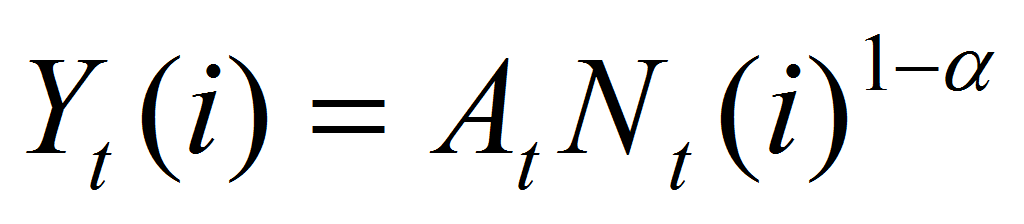, where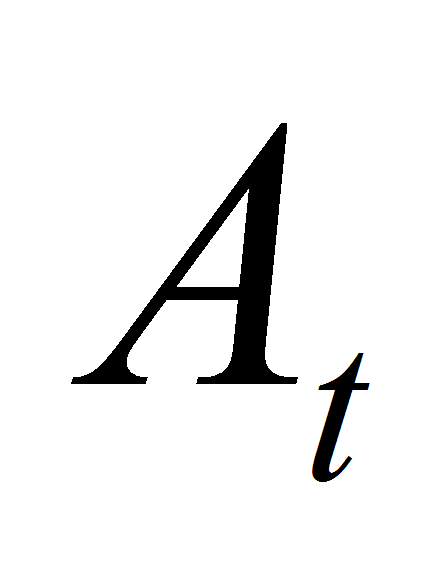represents the total factor productivity, which is identical for all firms.

In logs, the relation between output, employment and technology is described by the following log-linearized Cobb-Douglas production function, as follows: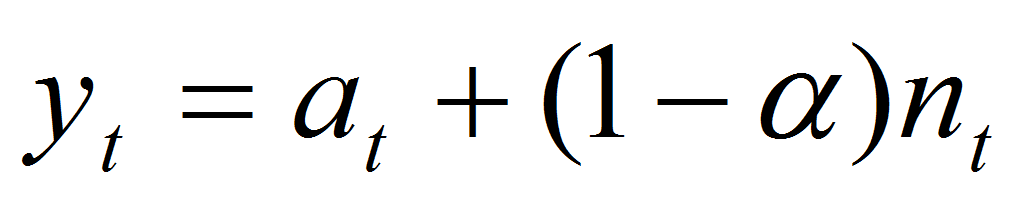.

Evidence on the effects of technology shocks and its implications are similar to those proposed by Gali, J. (1999) and Basu, Fernald and Kimball (2004), among others. Recent evidence, as well as alternative interpretations are surveyed in Gali and Rabanal (2004).

At one moment t,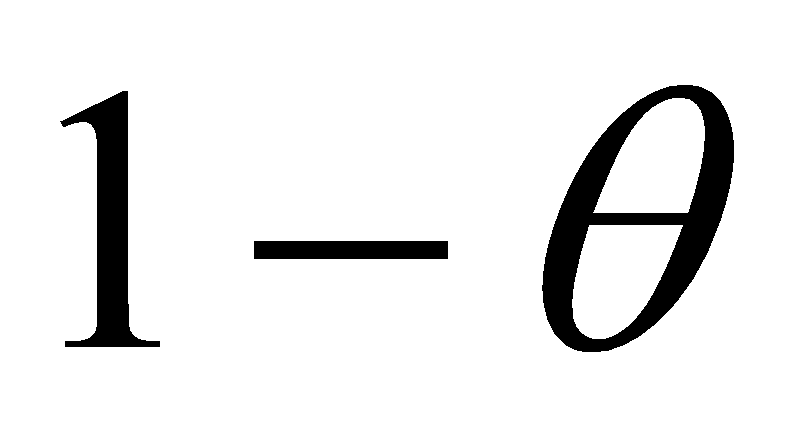of firms reset their price, while the remainingkeep the price unchanged.

The aggregate price dynamic is as follows: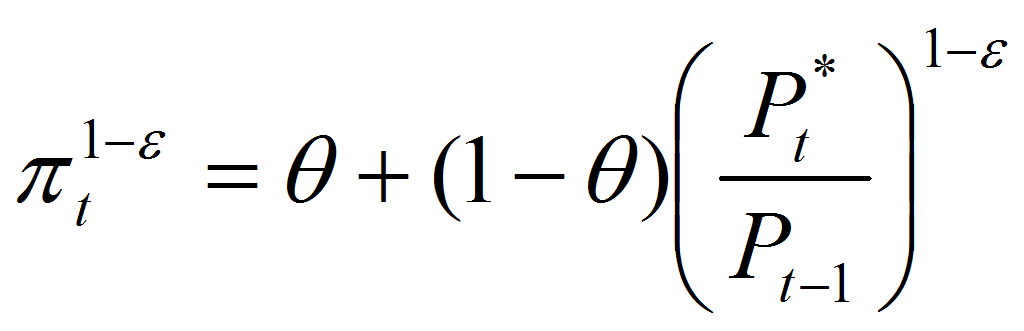, while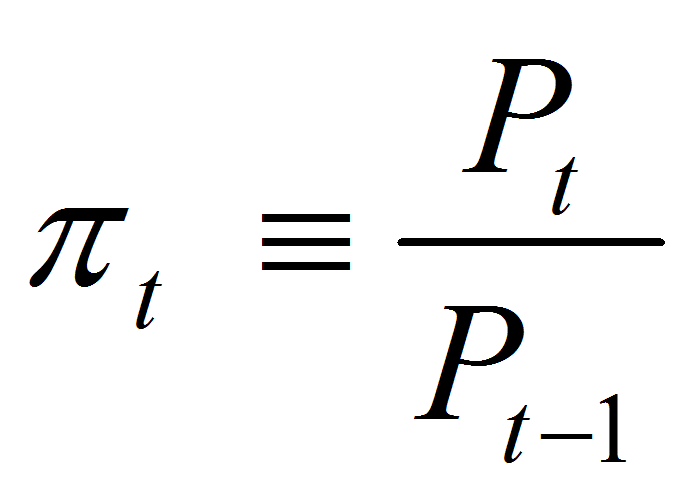defines inflation rate and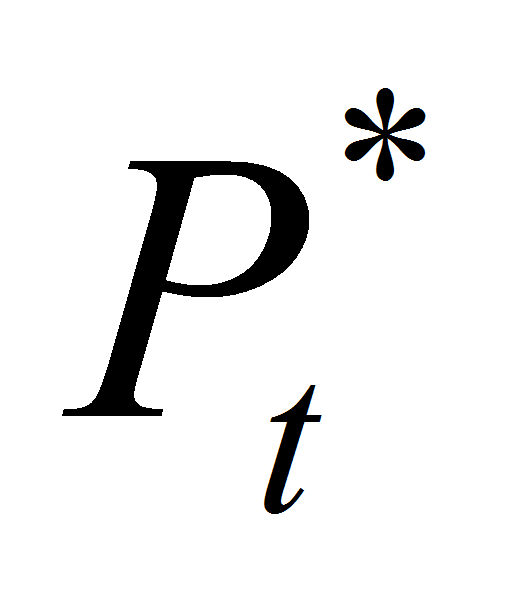represents the set price by firms reoptimizing. At steady-state, the equation becomes through log linearization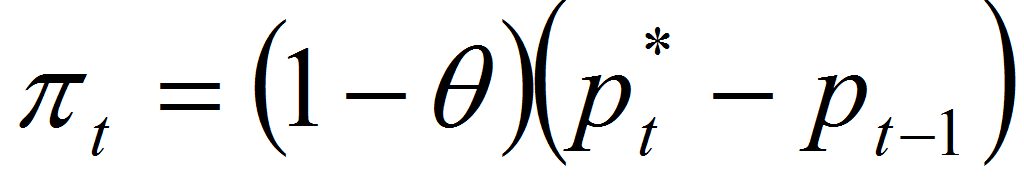.

For optimal price setting, the maximization function for firms that set optimal pricein order to maximize the profit is: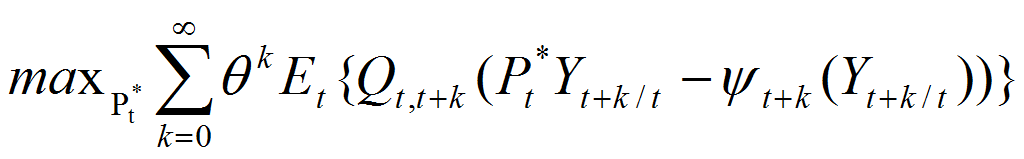subject to the sequence of demand constraints: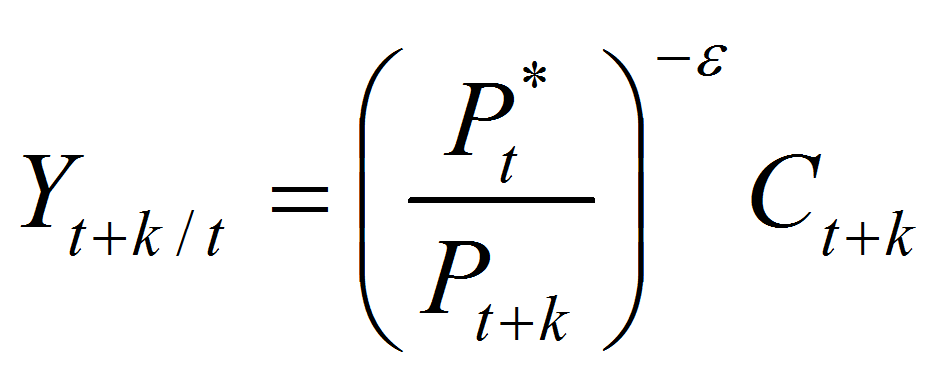, where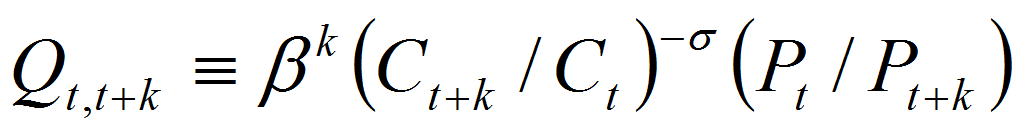represents the stochastic discount factor of payoff,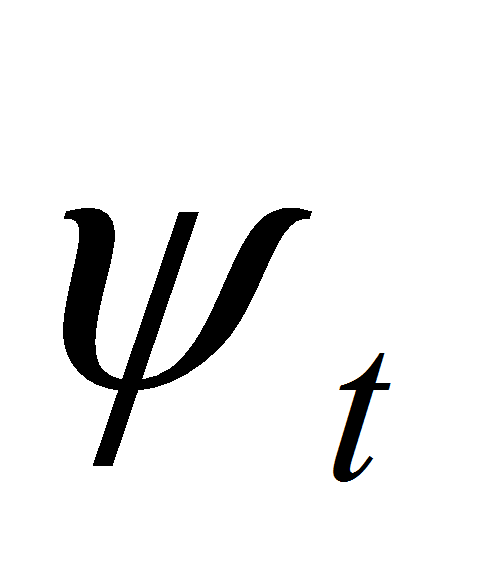is the cost function and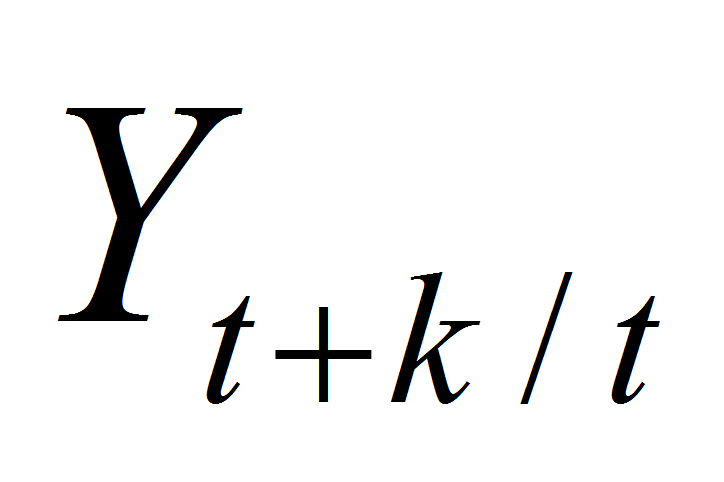represents the output in period t+k for firms resetting the price in period t. The resulting log-linearized form of maximization function is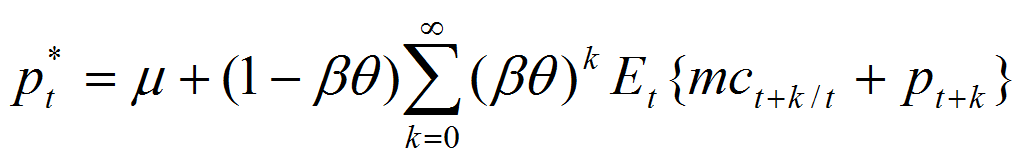, where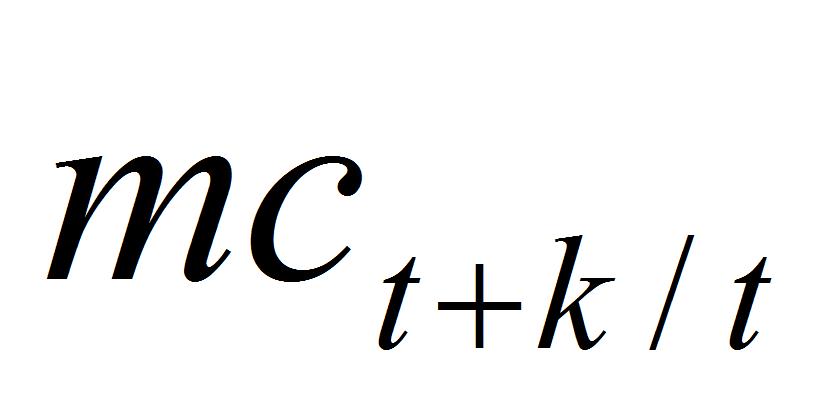represents the log of marginal cost andis the desired gross mark-up.

At equilibrium market clearing condition requires that supply is equal with demand,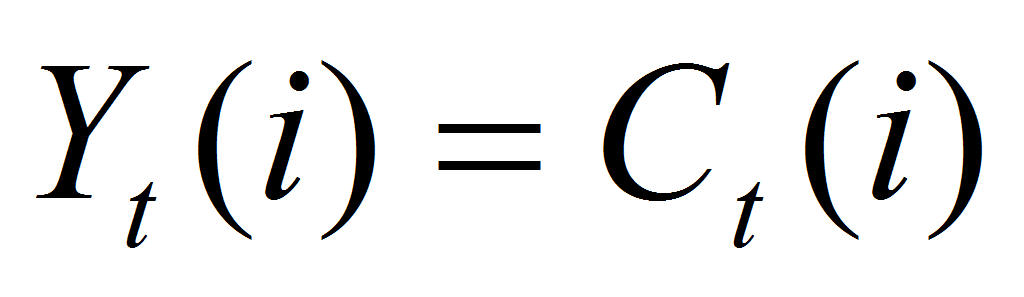(and respectively,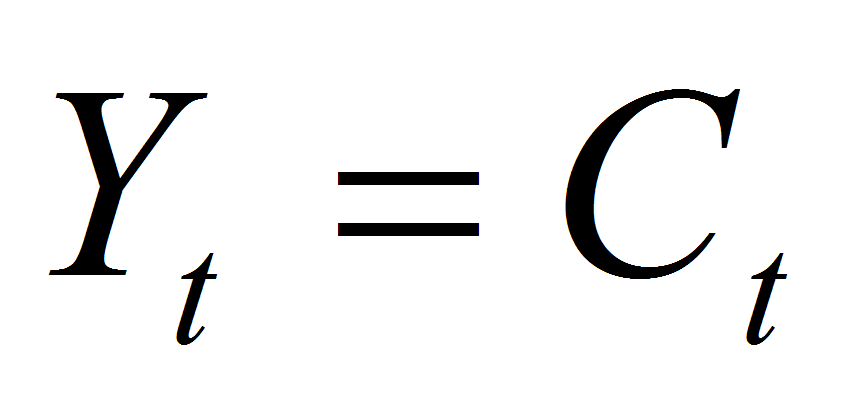in aggregate), where the aggregate output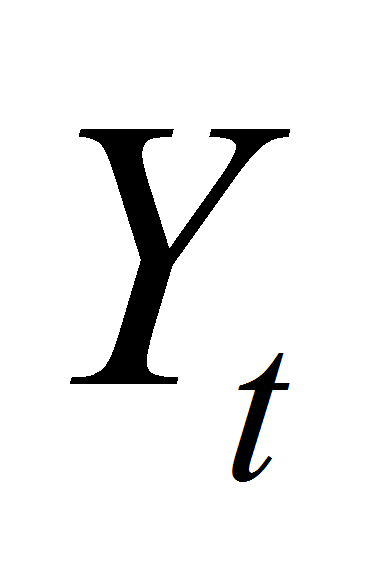is composed of a continuum of intermediate goods,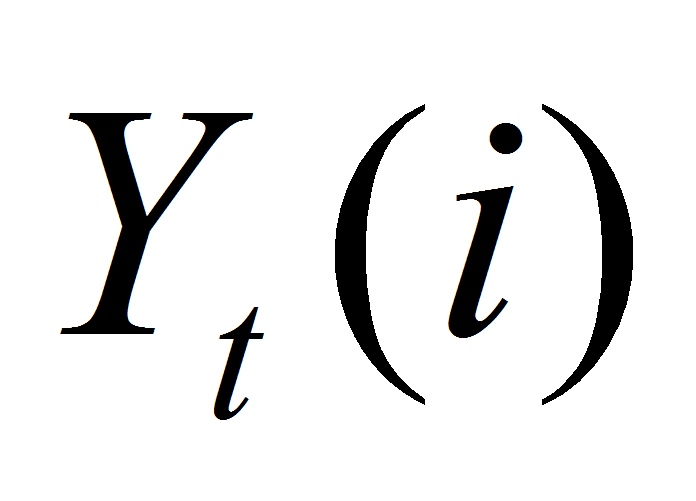as in the working paper of Kimball (1995), described as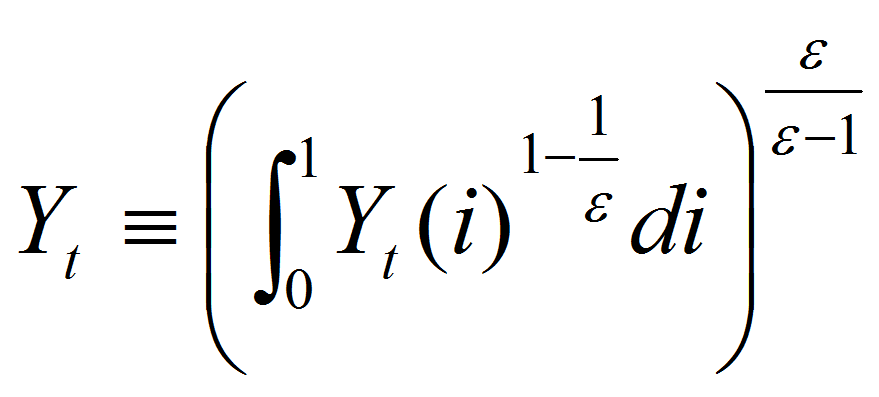.

From market clearing condition and consumer’s Euler equation, the resulting equilibrium condition is: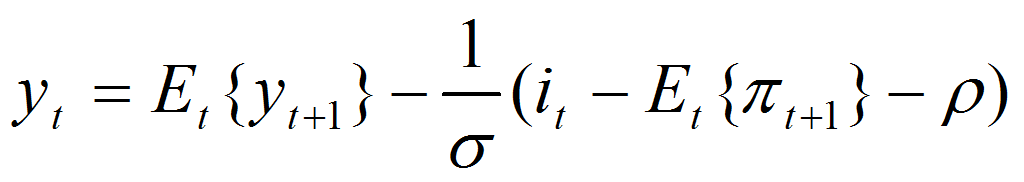.

Market clearing in case of labor market is: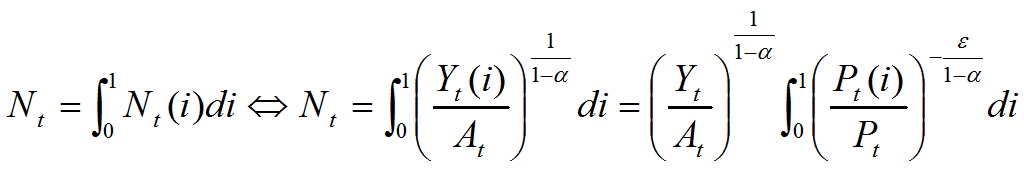.

Inflation equation is described as follows: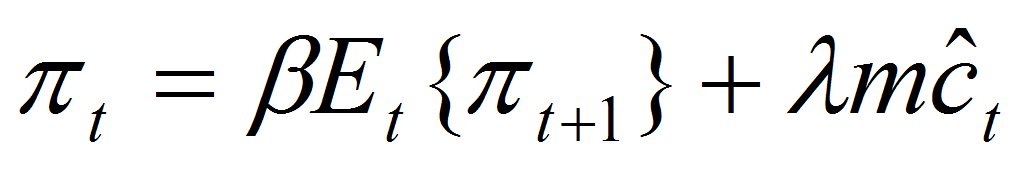, where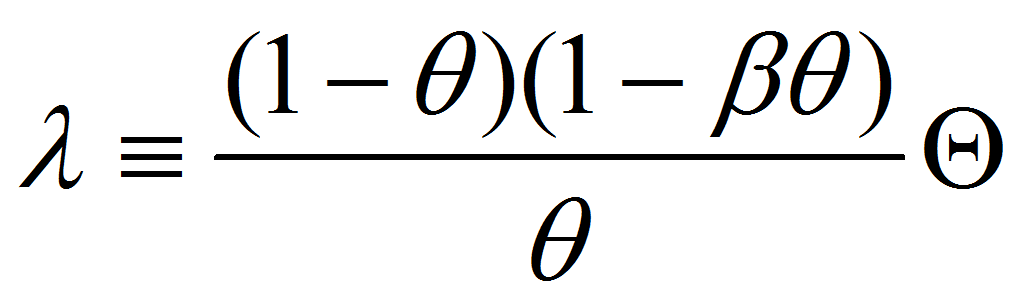and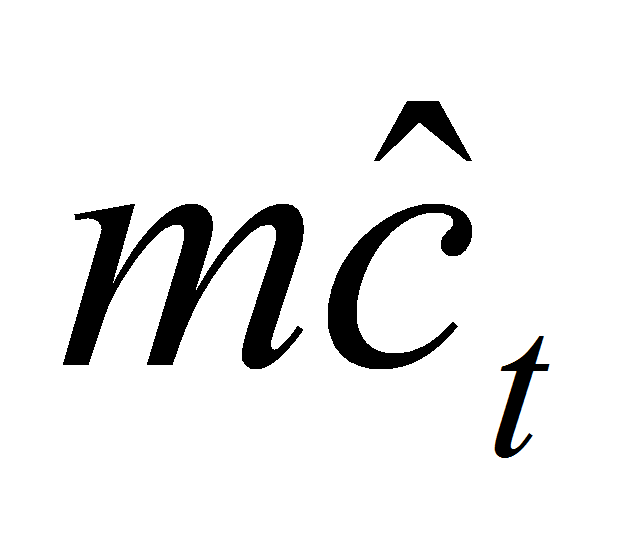is the deviation from steady state of real marginal cost.

The derived equation relating inflation to output gap and inflation from t+1 is described by the following New Keynesian Philips curve: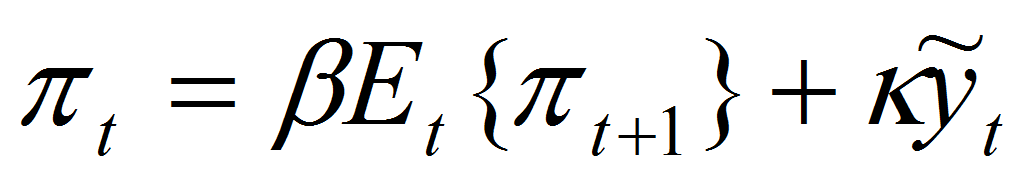, where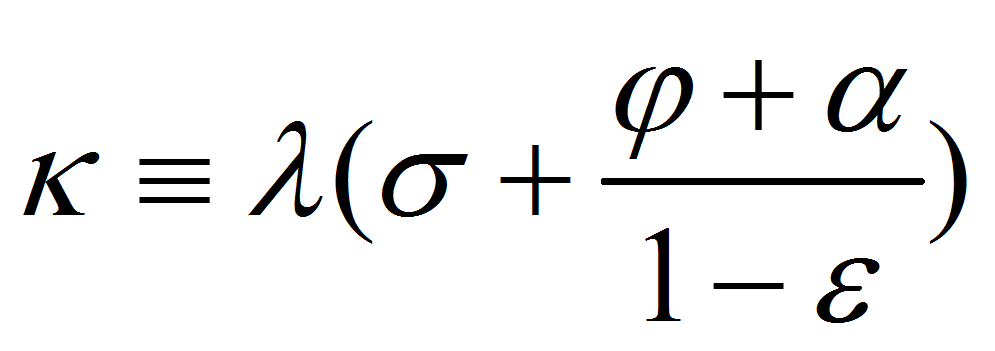.

An inflation equation identical to the New Keynesian Phillips curve can be derived under the assumption of quadratic costs of price adjustment, as shown in Rotemberg, J. (1982).

The equation of equilibrium related to the output gap for the New Keynesian model is described as follows: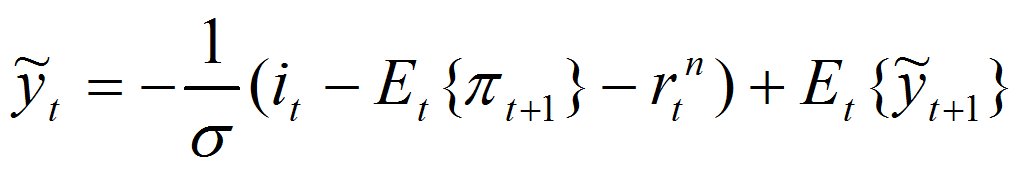, where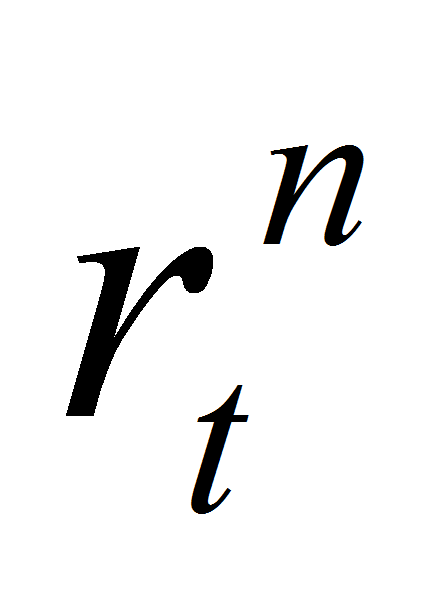is the natural interest rate, defined as follows: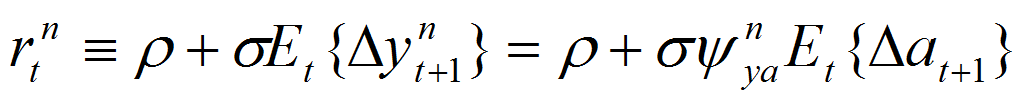.

3. Equilibrium Dynamics under Monetary Policy Rule

The current article proposes the analysis of equilibrium under a simple monetary policy rule of the form: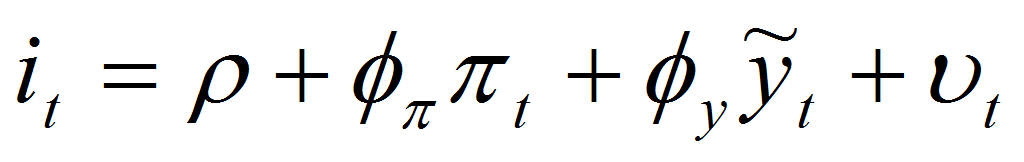, where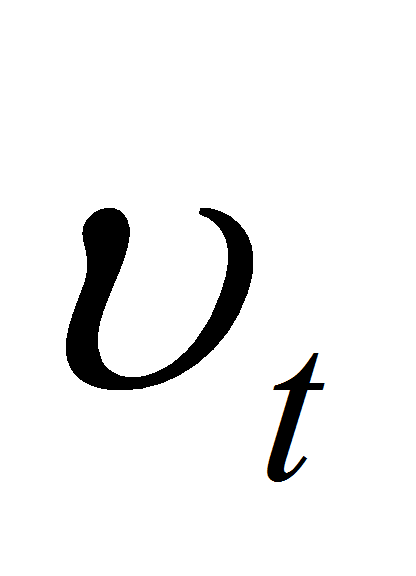is an exogenous component with zero mean,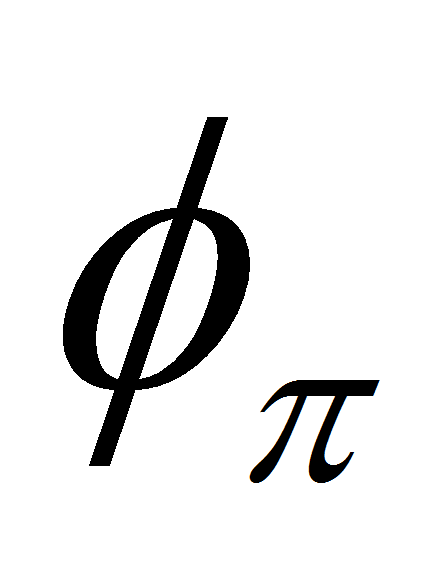and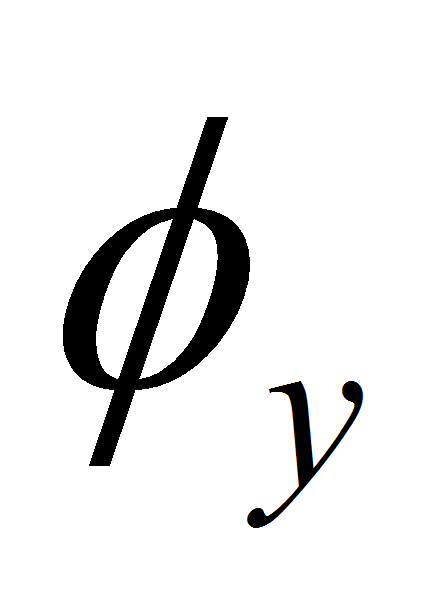are non-negative coefficients, chosen by monetary authority andis a constant factor.

The equilibrium conditions result from the following system of difference equations: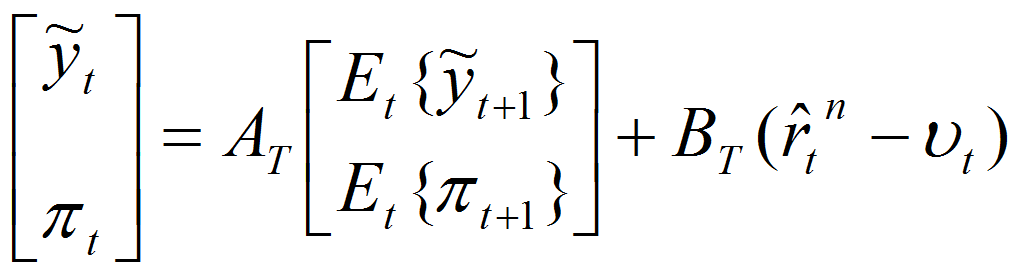, where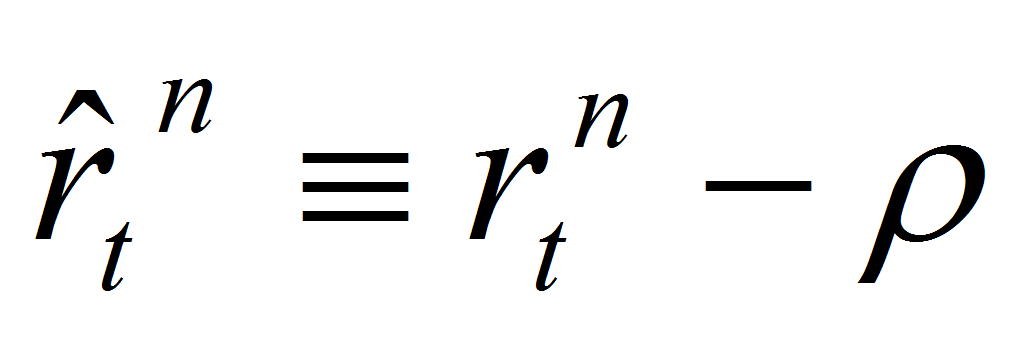and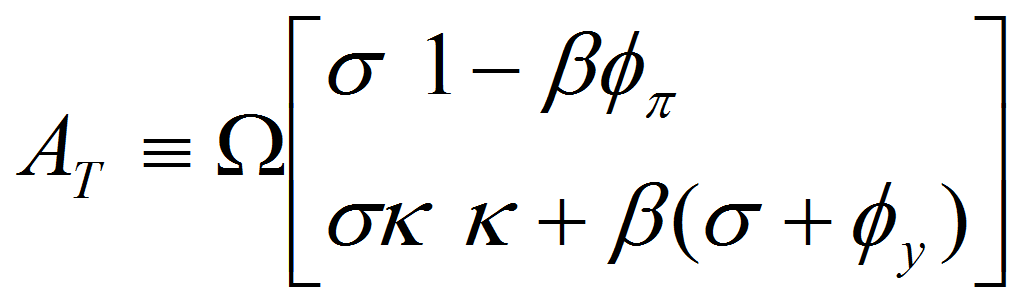;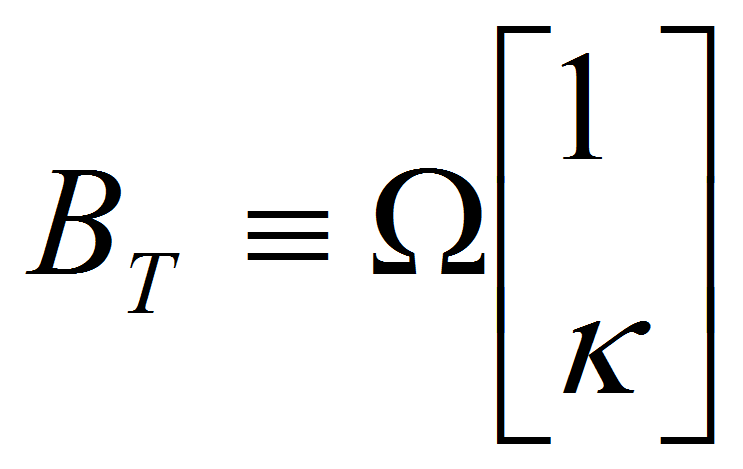and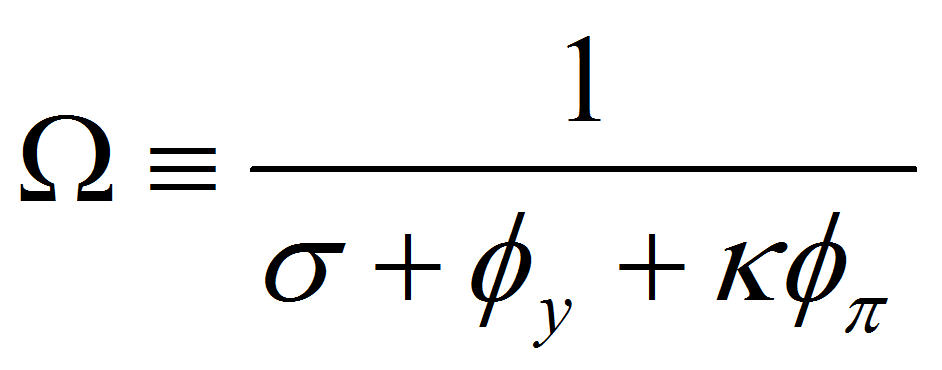.

3.1. Model Summary

The analyzed model consists of a total number of 25 variables, out of which number of state variables is 6 (variables for which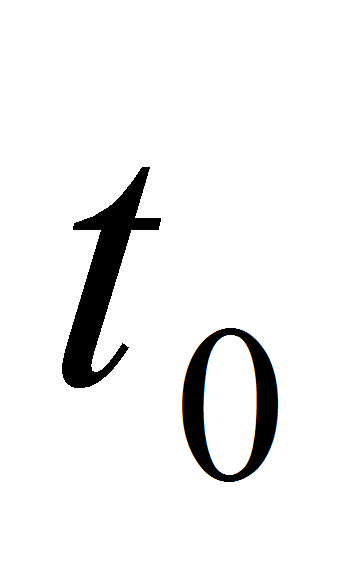moment is defined by the model) and the number of control variables is 19 (of which 2 forward looking variables- which appear in equations of the model at “t+1” moment and 17 static variables- which appear in equations of the model at “t” moment). The number of stochastic shocks is 3.

The variables of the model are: inflation, output gap, natural output, output, output deviation from steady state, natural interest rate, real interest rate, nominal interest rate, number of hours worked, real money stock, money growth, money growth annualized, nominal money stock, annualized real interest rate, annualized nominal interest rate, annualized natural interest rate, annualized inflation rate, price level, nominal wage, real wage, consumption, price markup and mark-up gap, monetary policy shock factor and technology shock factor.

All model variables are expressed in deviations from steady state and at steady-state all variables are 0 due to model linearization.

The three shocks of the model are: monetary policy shock, technology shock and preference shock.

3.2. Calibration and Prior Distribution of the Parameters

One important step of estimation of a DSGE model consists of calibration of the model’s parameters. As part of the strong econometric approach of estimation, as I used for the current model, the Bayesian approach by using the likelihood function with prior distributions for the parameters of the model, in order to form the posterior distribution. This posterior is afterwards optimized with respect to the model parameters either directly or through Monte-Carlo Markov- Chain (MCMC) sampling methods, as in F.Canova, (2007).

For the calculation of the likelihood function of the observed data series, I have used the Kalman filter, as in Sargent T.J. (1989).

In terms of parameterization most of these parameters are directly related to the steady-state values of the state variables and could therefore be estimated from the means of the observable variables. The standard errors of the innovations are assumed to follow an inverse-gamma distribution with two degrees of freedom.

The quarterly discount factor,, is set at 0.99, based on the average rate of 3M -5M bonds issued by state, implying a real return on financial assets of about 4%. The log utility parameter is assumed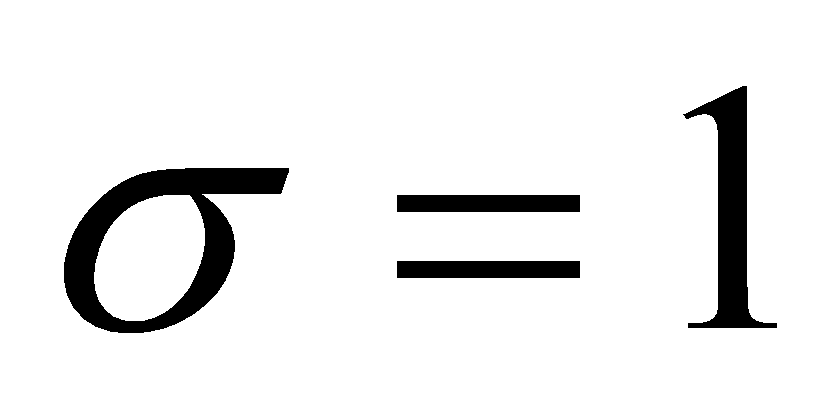and=1 (labour supply elasticity),=1/3 and=6, according with the common literature.

The money demand semi-elasticity to the interest,, is established at 4,indicator of price stickiness is set at 2/3, resulting an average contract price duration () of three quarters. In term of monetary policy rule coefficients, the coefficient of target inflation and of output gap are set as:=1.5,=0.125, while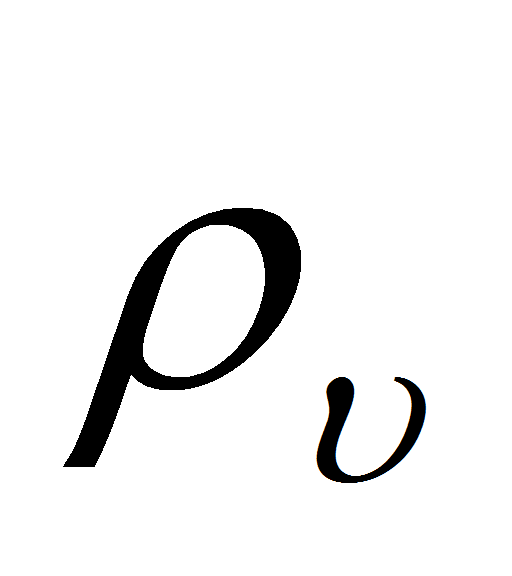=0.5 represents a moderate persistent interest rate shock.

4. Results

4.1. Equilibrium under an Interest Rate Rule

The analysis of matrix covariance indicates a zero covariance of the three shocks: monetary policy shock, technology shock and preference shock and the standard deviation of preference or discount rate shock.

 Variables eps_a eps_nu eps_z eps_a 0 0 0 eps_nu 0 0.0625 0 eps_z 0 0 0

We have also analyzed the historical variance decomposition of the endogenous variables. Table 2 below illustrates the deviation of the smoothed value of the endogenous variables from their steady state for the specified set of parameters, without taking into consideration the steady-state, based on the contribution of the smoothed shocks to the deviation of smoothed endogenous variables from steady state.

As resulted from the variance decomposition (table 2 below), the most significant shock that influences the each variable is considered the monetary policy shock, in case of equilibrium under an interest rate rule.

Table 2. Variance decomposition (%) Table 3. Coefficients of autocorrelation

 eps_a eps_nu eps_z Order 1 2 3 4 5 y_gap 0 100 0 y_gap 0.5 0.25 0.125 0.0625 0.0313 pi_ann 0 100 0 pi_ann 0.5 0.25 0.125 0.0625 0.0312 Y 0 100 0 Y 0.5 0.25 0.125 0.0625 0.0313 N 0 100 0 N 0.5 0.25 0.125 0.0625 0.0313 w_real 0 100 0 w_real 0.5 0.25 0.125 0.0625 0.0313 i_ann 0 100 0 i_ann 0.5 0.25 0.125 0.0625 0.0313 r_real_ann 0 100 0 r_real_ann 0.5 0.25 0.125 0.0625 0.0312 Nu 0 100 0 Nu 0.5 0.25 0.125 0.0625 0.0312

The coefficients of autocorrelation (table 3 above) indicate the lack of autocorrelation for the analyzed variables from first order to the 5th order.

In terms of stability of the system of equations, the rank condition this is verified, as there are 2 eigenvalues larger than 1 in modulus for 2 forward-looking variables, which means that the Blanchard-Kahn condition is met.

4.2. The Effects of a Monetary Policy Shock

The exogenous interest rate component,has the form of a process AR(1):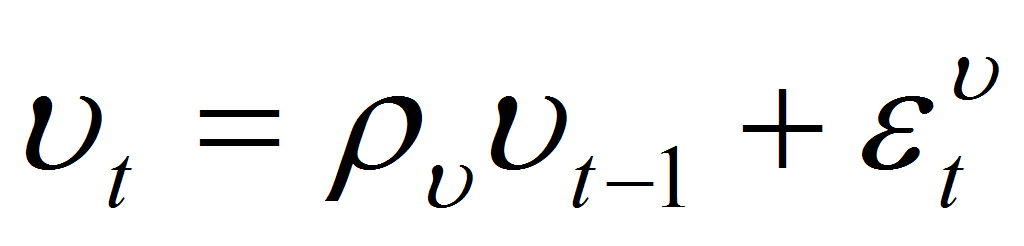, where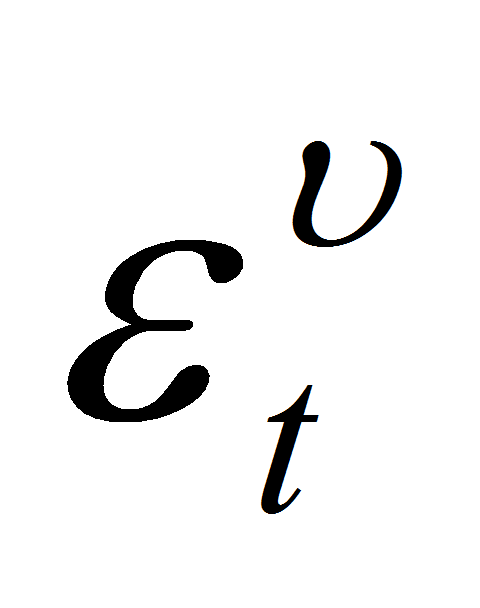indicates the tightening or expansionary monetary policy shock, leading to a rise or decrease of nominal interest rate, given inflation and output gap.

An exogenous increase of the interest rate as results from graph 1 below leads to a persistent decrease of the output gap and inflation. As the natural level of output is not influenced by the policy shock, the response of output matches that of the output gap. The response of the nominal interest rate includes the direct effect ofand the variation induced by lower output gap and inflation.

If the persistence of the monetary policy shockis sufficiently high, the nominal rate will decrease in response to a rise in, as a result of the downward adjustment in the nominal rate induced by the decline in inflation and the output gap more than offsetting the direct effect of a higher. As a result, despite the lower nominal rate, the policy shock still has a tightening effect on output, because it is inversely related to the real rate. The figure below represents the dynamic response of an expansionary monetary policy shock of an increase of one standard deviation (25 basis points) in. This shock, without further change induced by the response of inflation or the output gap, would imply an increase of 100 basis points to the annualized nominal rate.

Moreover, the policy shock generates an increase in the real rate and a decrease of inflation and output and, as a result, central bank has to reduce the money supply.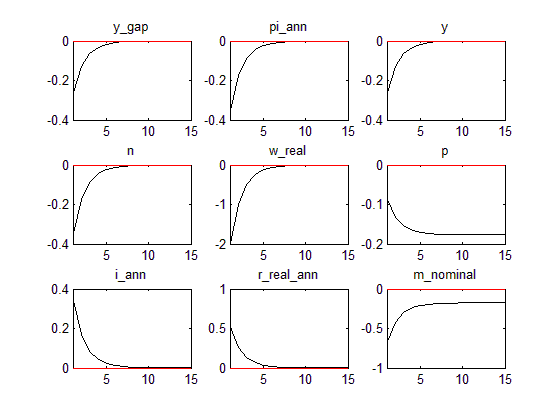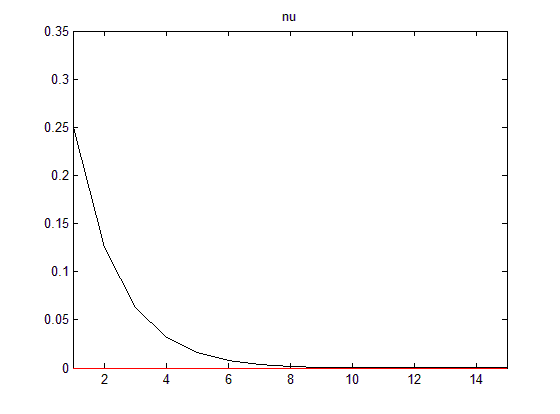Graph 1. IFR of a monetary policy shock Graph 2. Ortogonalized monetary policy shock

As results from the above graph, a negative monetary policy shock of one standard deviation in case of(meaning decrease of the interest rate) as part of an expansionary monetary policy leads a decrease of inflation, wages and of GDP (as a result of the output gap decrease) and finally leads to an increase of the nominal interest rate.

Moreover, the impact of an expansionary monetary policy is the increase of real interest rate, having an impact higher than in case of nominal interest, due to further decrease in expected inflation.

As a result, the authority responsible with the monetary policy should intervene in order to decrease the nominal interest rate, through another monetary policy instrument, by decreasing money supply.

The decomposition of orthogonalized shock as described in graph 2 above is indicated in case of inter-correlated shocks, but the graph analysis is even relevant in case shocks are not correlated. The graphical analysis indicates the responses of GDP variable as a result of a monetary policy shock, without including the effects it has on the rest of the variables in the system, in order to analyze the impact of one single shock at one moment of time, to one single variable. In case of an uncorrelated (orthogonal) monetary policy shock, as described in graph 2 above, the interpretation is that a change in interest rate shock with the size of one standard deviation has an isolated effect of increase with 0.25% of interest rate in log deviation percentages (on OY axis).

4.3. The Effects of a Technology Shock

The technology parameter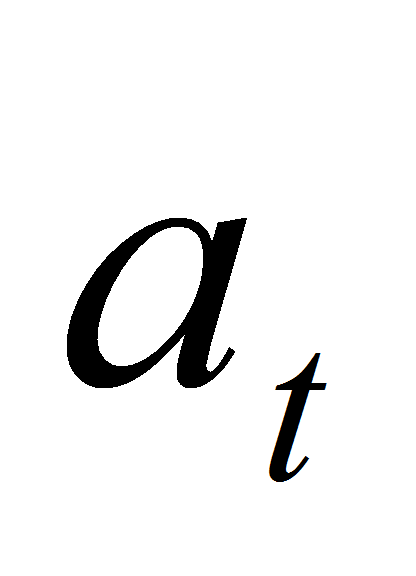follows the following AR (1) process: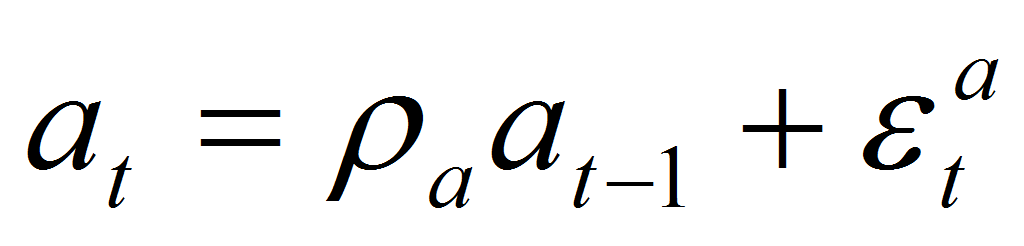According with the graph 3, a positive technologic shock,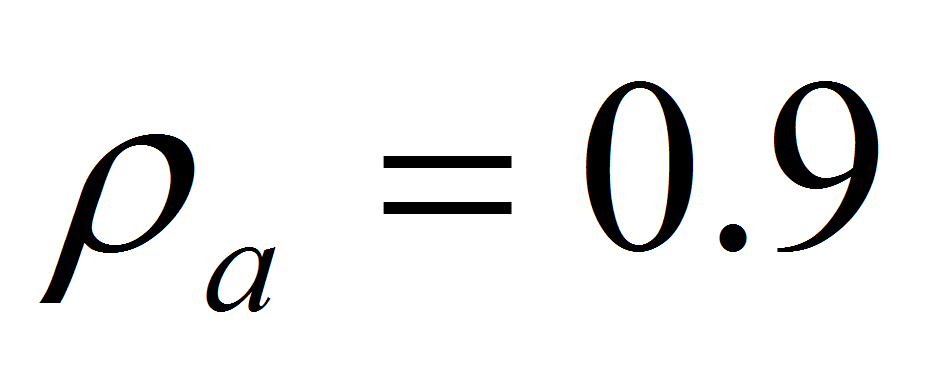leads to a persistent employment decrease. Moreover, as a result of a positive technologic shock Central Bank diminishes the interest rate and increases the money supply, as part of an expansionary monetary policy. Output increases while employment and real wages decrease. Moreover as can be seen from the graph below, the output gap and inflation fall below zero steady-state.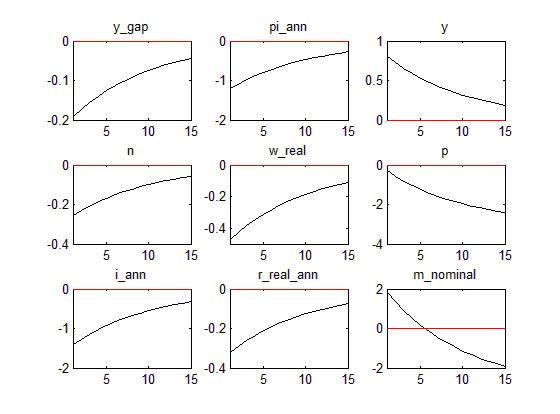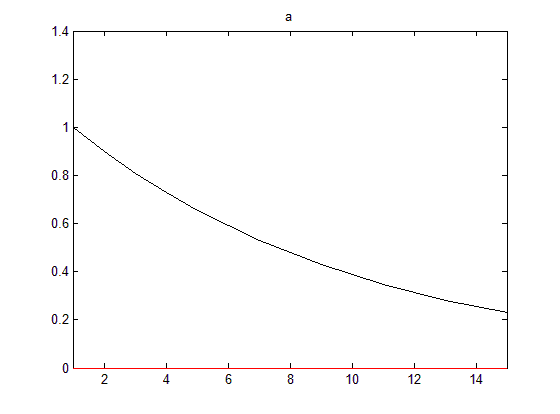Graph 3. IFR for a technology shock Graph 4. Orthogonalized technology shock

In case of an uncorrelated (orthogonal) technology shock, as described in graph 4 above, the interpretation is that a change in technology shock with the size of one standard deviation has an isolated effect of increase with 1% of GDP in log deviation percentages (on OY axis).

4.4. The Effects of a Preference Shock

In case of a positive preference shock as results from the graph 6 below there is a decrease of output (influenced by the decrease of output gap), decrease of inflation, of real wages, of real interest rate, of employment and a final decrease below steady-state of the nominal interest rate.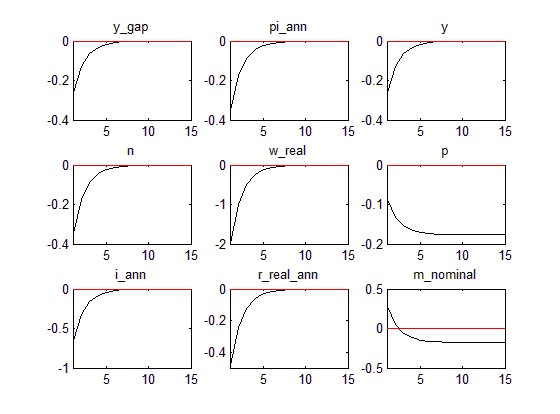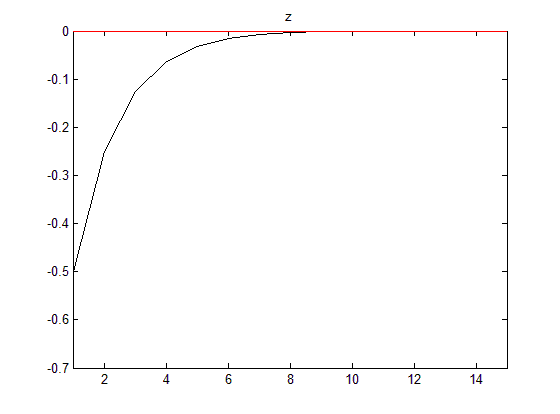Graph 5. IFR for a preference/discount rate shock Graph 6. Orthogonalized preference shock

In case of an uncorrelated (orthogonal) preference shock, as described in graph 6 above, the interpretation is that a change in preference shock with the size of one standard deviation has an isolated effect of decrease with 0.5% of GDP in log deviation percentages (on OY axis).

5. Conclusions

The New Keynesian model proposed for analysis indicates the significance of the exogenous shocks such as: monetary policy shock, technology shock and preference shock on the behavior of macroeconomic variables such as GDP, as economic welfare indicator, of employment index, inflation, real wages and also on interest rate and money supply, as monetary policy instruments.

The analysis if focused on the impact of conducting macroeconomic policy through the Taylor rule proposed in relation with the welfare analysis, in the context of uncertainty affecting the stochastic behavior of the macroeconomic variables through exogenous shocks.

The model is validated by the results obtained and also by the tests performed, such as: Blachnard-Kahn stability test performed for the equation of the system, analysis of the coefficients of autocorrelation, orthogonalized shocks and variance decomposition. In addition, the results for the impulse response functions for the three shocks are in line with macroeconomic evidence: an expansionary monetary policy leads to a decrease of GDP, wages and inflation and finally lead to an increase of the nominal and real interest rate. As a result, the authority responsible with the monetary policy should intervene in order to decrease the nominal interest rate by decreasing money supply.

In case of a positive technologic shock central bank diminishes the interest rate and increases the money supply, as part of an expansionary monetary policy. Output increases while employment, real wages decrease, output gap and inflation fall below steady-state. In case of a positive preference shock there is a decrease of output (influenced by the decrease of output gap), decrease of inflation, of real wages, of real interest rate, of employment and a final decrease below steady-state of the nominal interest rate. To conclude, the model proposed by the current working paper has achieved the purpose to analyze the impact of exogenous shocks to the macroeconomic variables, in the context of a monetary policy rule based on targeting inflation and output gap.

6. References

Basu, S.; Fernald, J. & Kimball, M. (2004). Are Technology Improvements Contractionary?. American Economic Review, 96, no. 5, pp. 1418–1448.

Canova, F. (2007). Methods for Applied Macroeconomic Research. New Jersey: Princeton University Press, pp. 1-45.

Gali, J. (2015). Monetary Policy, Inflation, and the Business Cycle: An Introduction to the New Keynesian Framework and Its Applications (Second Edition). Princeton University Press, pp. 41-70.

Gali, Jordi & Rabanal, Pau (2004). Technology Shocks and Aggregate Fluctuations: How Well Does the RBC Model Fit Postwar U.S. Data?. NBER Macroeconomics Annual 2004, pp. 225–288.

Gali, J. (1999). Technology, Employment, and the Business Cycle: Do Technology Shocks Explain Aggregate Fluctuations? American Economic Review, 89, no. 1, pp. 249–271.

Kimball, M. (1995). The quantitative analytics of the basic neo-monetarist model. Journal of Money, Credit and Banking, 27(4), pp. 1241-1277.

Rotemberg, Julio (1982). Monopolistic Price Adjustment and Aggregate Output. Review of Economic Studies, 49, pp. 517-531.

Sargent, T.J. (1989). Two models of measurements and the Investment Accelerator. Journal of Political Economy, 97:2, pp. 251-287.

1 PhD Student, Academy of Economic Studies, Doctoral School of Finance and Law, Bucharest, Romania, Address: 6 Piata Romana, Cladirea Ion N. Angelescu, Camera 0327, Romania, Tel.: +4021 319 1900, Corresponding author: Georgiana.alina.ionita@gmail.com.

AUDŒ, Vol. 13, no. 1, pp. 5-16

### Refbacks

• There are currently no refbacks.